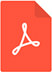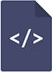Optimization of distance between fire stations: effects of fire ignition probabilities, fire engine speed and road limitations, property values and weather conditionsPDF Full Text

Abstract

A general spatial fire brigade unit network density optimization problem has been solved. The distance to a particular road, from a fire station, is approximated as a continuous variable. It is proved, via integral convolution, that the probability density function of the total travel time, PDFT, is triangular. The size of the fire, when it stopped, is a function of the time it takes until the fire brigade reached the fire location. An explicit continuous function for the expected total cost per square kilometer, based on the cost per fire station, the PDFT, the exponential fire cost function parameters, the distance between fire stations, and the speed of fire engines, is derived. It is proved that the optimal distance between fire brigade unit positions, OFD, which minimizes the total expected cost, is unique. Then, the OFDs are replaced by integers, OFDIs, for different parameter assumptions. In this process, also the optimal expected total costs are determined. It is proved that the OFD is a strictly decreasing function of the expected number of fires per area unit, a strictly increasing function of the speed of the fire engines, a strictly decreasing function of the parameters of the exponential fire cost function, and a strictly increasing function of the cost per fire station. These effects of parameter changes are also illustrated via graphs in the numerical section.